# Frequency of Compounding

In the illustrations of the present value of 1 in Part 1 we assumed that interest was compounded on an annual basis. Now we'll look at what happens when interest is compounded (1) annually, (2) semiannually, (3) quarterly, and (4) monthly.

The tables below show the number of periods (n) and the related interest rate (i) for four different compounding assumptions.

The following table reflects a single amount invested for one year and earning an annual interest rate of 12%: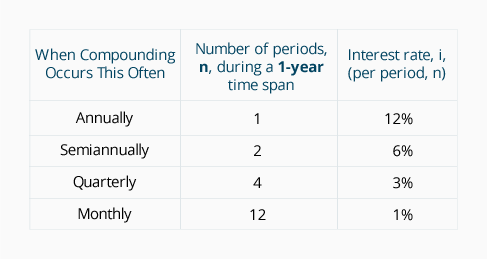The following table reflects a single amount invested for two years and earning an annual interest rate of 12%: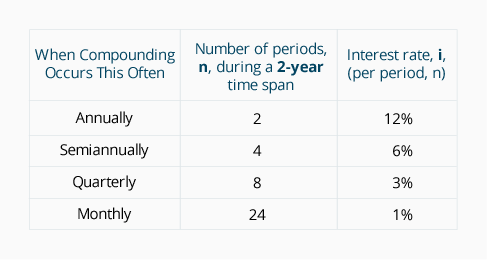To demonstrate the variations that occur with different compounding assumptions, let's look at an example: Assume that on January 1, 2021, a single deposit of \$10,000 was made into each of four accounts. Although each of the four accounts earns an annual interest rate of 12%, the interest is compounded differently. We'll track the account activity for two years.

1. Compounded annually
Since our first account is compounded annually, it receives two interest deposits—one at the end of each year; we refer to this as n = 2. The annual interest rate is 12%; we refer to this as i = 12% per year.

Here's how the account activity will occur: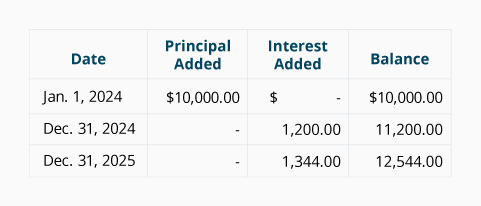At the end of 2021, \$1,200 of interest had been earned on the balance of \$10,000; this interest was automatically reinvested and added to the balance. In 2022, \$1,344 of interest was earned on a balance of \$11,200 (\$1,200 on the principal of \$10,000 plus \$144 on the \$1,200 of interest earned in 2021.)

2. Compounded semiannually
Our second account is compounded semiannually and receives four interest deposits—one at the end of each six-month period. If we view the annual interest rate of 12% as a semiannual interest rate of 6%, it means that the two-year investment will have n = 4 semiannual interest deposits, and i = 6% per half-year. Here's how the account activity will occur: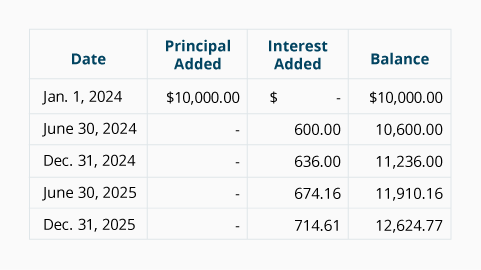3. Compounded quarterly
Our third account is compounded quarterly and receives eight interest deposits—one at the end of each three-month period. If we view the annual interest rate of 12% as a quarterly interest rate of 3%, it means that the two-year investment will have n = 8 quarterly interest deposits, and i = 3% per quarter. Here's how the account activity will occur: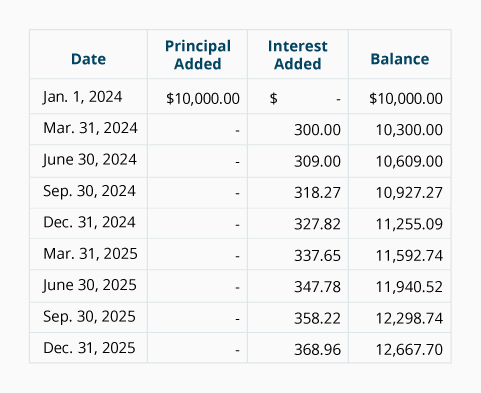4. Compounded Monthly
Our fourth account is compounded monthly and receives 24 interest deposits—one at the end of every month. If we view the annual interest rate of 12% as a monthly interest rate of 1%, it means that the two-year investment will have n = 24 monthly interest deposits, and i = 1% per month. Here's how the account activity will occur: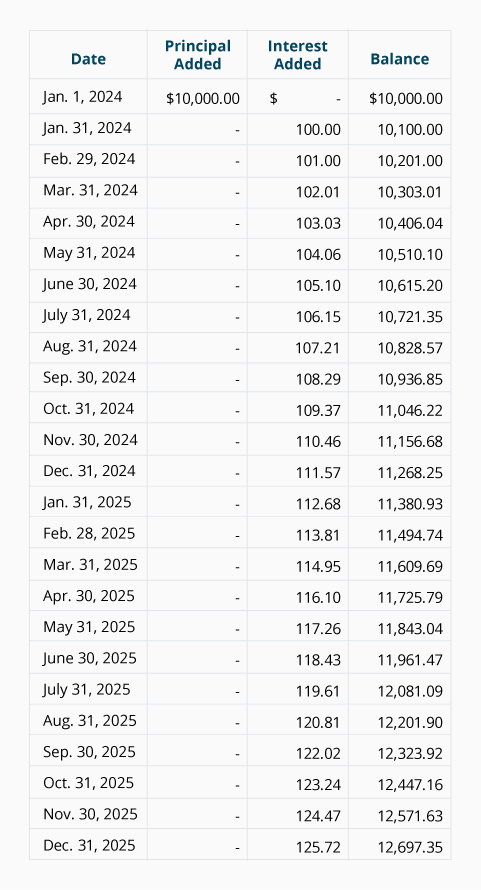The next two tables compare selected account balances from each of the four accounts above:

Table 1
Comparison of Balances in Accounts After One Year—at Dec. 31, 2021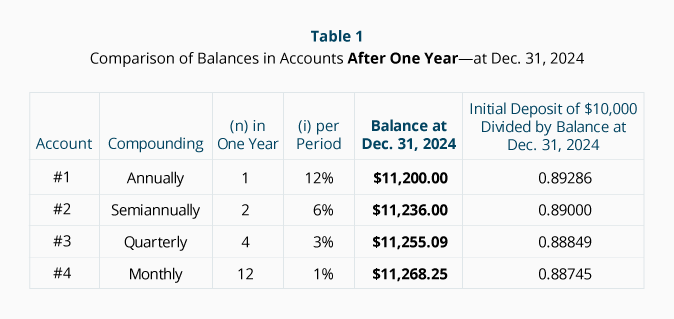Table 2
Comparison of Balances in Accounts After Two Years—at Dec. 31, 2022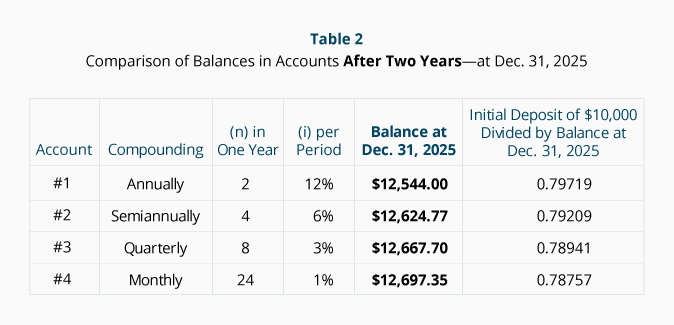As you have seen, the frequency of compounding requires you to adjust the number of periods (n). Likewise, the interest rate (i) must be adjusted to be compatible with (n).

Compounding affects both present value and future value. In Table 2 we see that on December 31, 2022, Account #4—the account that is compounded monthly—has the highest future value: \$12,697.35. When interest is deposited monthly, the account earns "interest on interest" more often. In the last column we see a present value factor of 0.78757—this tells us the amount we need in the present time to grow to 1.000 in the future. In other words, because of monthly compounding, we need only deposit \$0.78757 today for it to grow to be \$1.00 at the end of two years (with a 12% annual interest rate). With less compounding (e.g., annual, semiannual, or quarterly) we need to deposit more today in order for the amount to grow to \$1.00 during the same span of time as monthly compounding.

PV calculations greatly assist investment decisions because of their ability to bring future amounts into the context of the present (to time period 0). After all, it is hard to relate \$100,000 being spent today (a present value) to \$300,000 that is expected to be received 20 years from today (a future value). By discounting that future \$300,000 to a present value, we can more logically compare it to the \$100,000 because both amounts will be expressed in present value amounts.

## Handling More Than One Future Amount

If there are two or more future amounts occurring at different times for an investment, their present value can be determined by simply discounting each amount separately. For example, if an amount of \$5,000 occurs at the end of two years, and a second amount of \$6,000 occurs at the end of five years, you simply calculate the present value of each and combine them.

If the future amounts are identical in amount and they occur at equal time intervals, the situation is referred to as an annuity. (Learn more at Present Value of an Ordinary Annuity.)